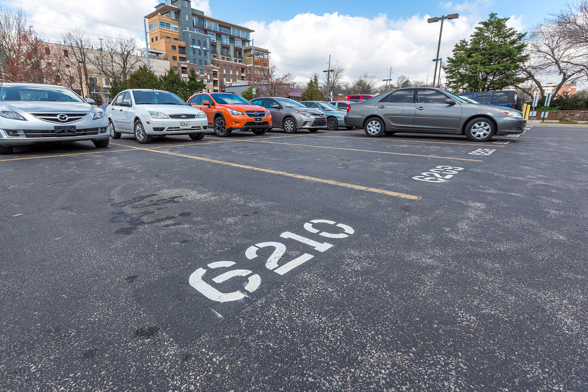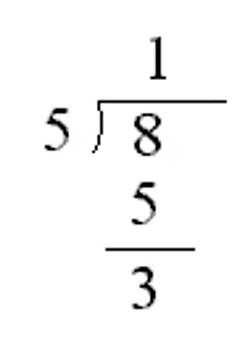# AP Java: Variables

## Variable types and sizes

Think of a variable as a place to store a value that you will use later. Variables get their name because they can vary or change. If you previously studied JavaScript or Python, you are going to notice something different about Java. Variables have different types and every Java type has size limits. For example, integers are limited to values between -2,147,483,648 and 2,147,483,647. Run the program below and you will get an error. The program is attempting to print the integer `123456789101112131415` which is too large.

A good way to think of Java variables is like a parking space. Parking spaces have different types that can accomodate different sized vehicles.For this class, you need to know 5 basic (also called primitive) types: ```int float double boolean ``` and `char`.

• `int` holds a single integer value between -2,147,483,648 and 2,147,483,647
• `float` a decimal value with up to about 7 digits
• `double` a decimal value with up to about 15 digits
• A `boolean` can only hold values that are either `true` or `false`
• `char` holds a single letter, digit, space or punctuation mark and must be enclosed in single quotes, like this: `'G'`

## Variable declarations

Parking spaces will contain different vehicles at different times, so some parking spaces are labeled to make it easier to find your parking space later. It's the same for Java variables. In addition to a type every Java variable has also has a name.The Java statement that names a variable is called a declaration. Here’s an example declaration

# `int num;`

The first word of the declaration is the type. The second word is the name that the programmer chooses. You can pretty much choose any name you want for a variable with a few limitations. Variable names cannot
• contain a space
• have a special meaning in Java
Java has about 50 reserved words or keywords that you are not allowed to use as variable names such as `public`, `class`, `static`, `void`, `int`

Because a Java variable name can’t have spaces, a style called camelCase is usually used for variable names with more than one word. camelCase capitalizes the first letter of each word except the first word like `firstName` or `numberOfDogs`. Java variable names are case-sensitive, so `firstName` is not the same as `firstname` or `FirstName`.

Here's some other example declarations using the other primitive types:

# `char letter;`

## Variable initializations

After a Java variable is declared, it needs to be initialized. Run the following program and you will get an error message.

The English word initial means first. We initialize a variable by assigning (“setting it equal to”) its first value. We can fix the error in the program by adding `num=3;` between lines 4 & 5. (Note it doesn't have to be `3`, we could use any integer between -2147483648 and 2147483647). Add an initialization between lines 4 and 5 and run the code again to verify that the error is fixed.

You can declare and initialize a variable with one line of code like

# `int num = 3;`

Just remember that the one line of code is doing two different steps: the declaration and the initialization

The following program has 5 errors because the 5 variables are initialized incorrectly. Fix the five initializations so that the program runs without error. Remember that

• `int` holds a single integer value between -2,147,483,648 and 2,147,483,647
• `float` a decimal value with up to about 7 digits
• `double` a decimal value with up to about 15 digits
• A `boolean` can only hold values that are either `true` or `false`
• `char` holds a single letter, digit, space or punctuation mark and must be enclosed in single quotes, like this: `'G'`

## Integer Division and Modulus

Java divides decimals differently that it does integers. Run the following program and look at the results.

You've already seen the operators `+ - * /`. There is another operater `%` called Modulus (also Mod or Modulo) that calculates the remainder of dividing two integers. Remember how you first learned division with a remainder in 3rd grade?Run the following program and you will see that the expression `8/5` evaluates to `1` with no decimals.

Now add two more lines of code under line 8 that print the remainder of 8 divided by 5.

The code.org modelo clock widget is a good tool for understanding modulus. You may find it helpful in figuring out the result of the following expressions.

• `12/5`
• `12%5`
• `23/3`
• `23%3`
• `6/7`
• `6%7`
• `6%10`
• `6%78912345`

Now write a program that displays the expressions and check your answers.

## Assignment: Write two programs `Initializations.java` and `Computus.java`

The following program has five error messages because the five variables have not been initialized. Initialize the variables so that the program runs without any error messages.

Write a second program that calculates and displays the date of Easter for a particular year. Easter Sunday is the first Sunday after the first full moon of Spring. The calculation of the date of Easter is called Computus in Latin. One algorithm for Computus was invented by mathematician Carl Friedrich Gauss in the early 1800s and is shown below. Your program will declare and initialize 14 `int` variables y, a, b, c, d, e, g, h, j, k, m, r, n, and p.

• Let y be the year.
• Divide y by 19 and call the remainder a. Ignore the quotient.
• Divide y by 100 to get a quotient b and a remainder c.
• Divide b by 4 to get a quotient d and a remainder e.
• Divide 8 * b + 13 by 25 to get a quotient g. Ignore the remainder.
• Divide 19 * a + b - d - g + 15 by 30 to get a remainder h. Ignore the quotient.
• Divide c by 4 to get a quotient j and a remainder k.
• Divide a + 11 * h by 319 to get a quotient m. Ignore the remainder.
• Divide 2 * e + 2 * j - k - h + m + 32 by 7 to get a remainder r. Ignore the quotient.
• Divide h - m + r + 90 by 25 to get a quotient n. Ignore the remainder.
• Divide h - m + r + n + 19 by 32 to get a remainder p. Ignore the quotient.
• The month that Easter falls on is n and the date is p. For example, if n is 4 and p is 15, Easter falls on 4/15 or April 15th of that year.

Test your program by using different years and googling the date of easter for that year. You can check your calculations with the following. For 2010 a, b, c, d, e, g, h, j, k, m, r, n, p should be 15, 20, 10, 5, 0, 6, 9, 2, 2, 0, 4, 4, 4.

Submit the links to your two finished programs by choosing Share | Link. Go to Google Classroom and and then choose Add Link for each program. Don't forget to click the Turn In button.